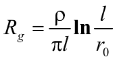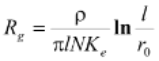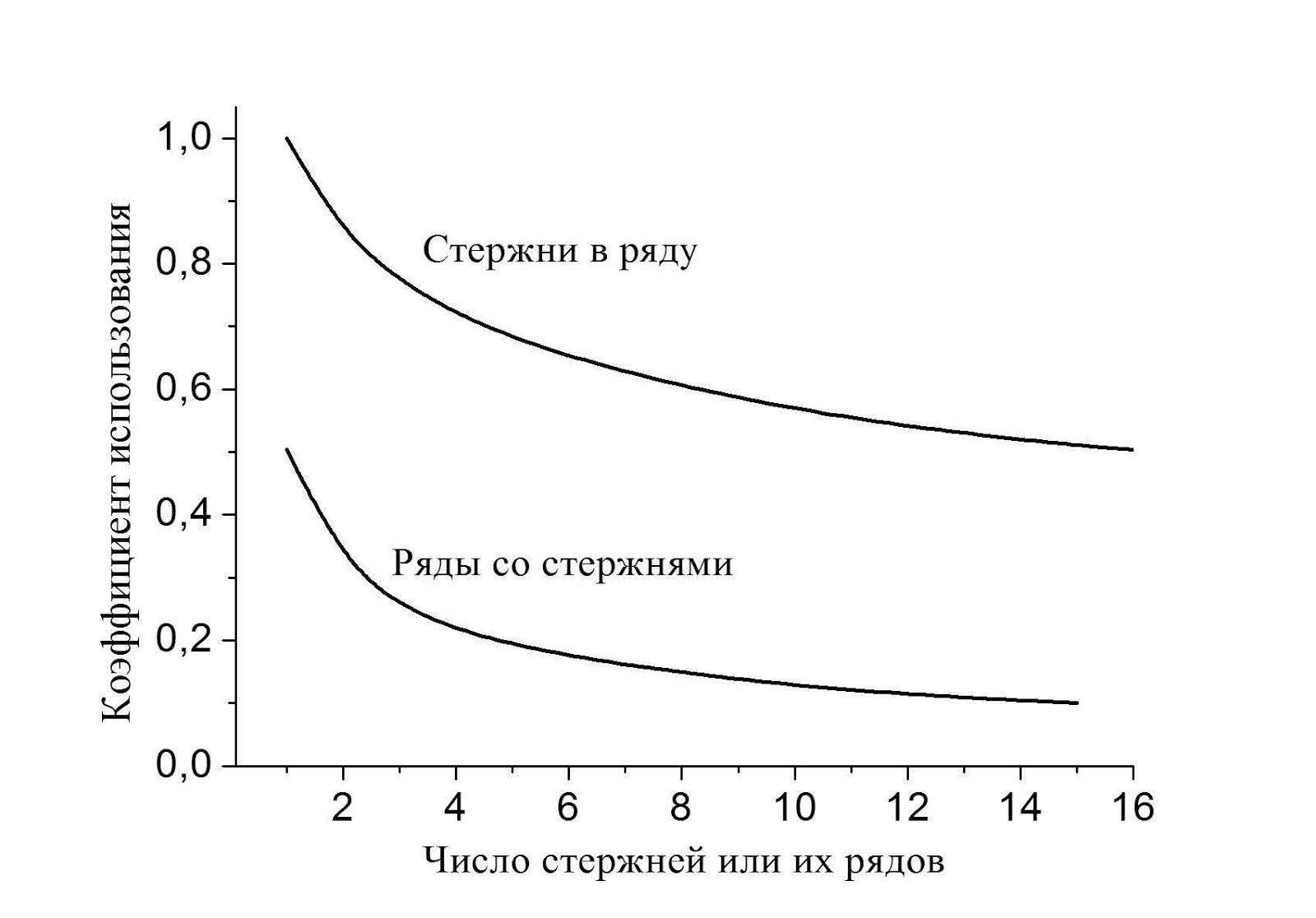# 1. Where did the load factors come from?

From the series of articles "Grounding resistance calculation is almost easy".

Imagine two vertical grounding electrodes of length l and radius r0. They are connected with a horizontal bus. Current, spreading in the soil, is supplied to the electrodes through the horizontal bus. The horizontal bus itself is in the air and is not connected to the ground. It seemed to someone that the vertical electrodes were connected in parallel. The grounding resistance of a single vertical electrode Rg in an unlimited amount of soil is well known. It can be found in any reference book. For now, in order to keep things easy, I will also use the reference book and write it out(1)

In this formula, ρ is the specific resistivity of the soil, and l and r0 are the length and radius of the electrode.

In our case, there are two electrodes. The resistance decreases inversely with the number of parallel identical conductors. It seems that the grounding resistance should also be halved, and for N identical electrodes – for N times. This conclusion is easy to verify by direct experimentation. Unfortunately, the verification will fail. The decrease in grounding resistance occurred, but to a much smaller extent. It turned out that the electrodes seemed to interfere with each other.(2)

I do not want to disclose a method for determining load factors for now, but I will show their absolutely strict values using an example of a series of rods that are driven into the ground in a straight line with a step of 3 m to a depth of 5 m. And for a system of parallel lines with the same clogged rods, let there be 15 on each parallel line, and the lines go with the same step of 3 m.Стержни в ряду - Rods in the row

Ряды со стержнями - Rows with the rods

Число стержней или их рядов - Amount of rods or their rows

Correcting the calculated value by 2 times can take place, but adjusting the initial value by 10 times can only speak of a complete methodological dead end.

Related Articles: